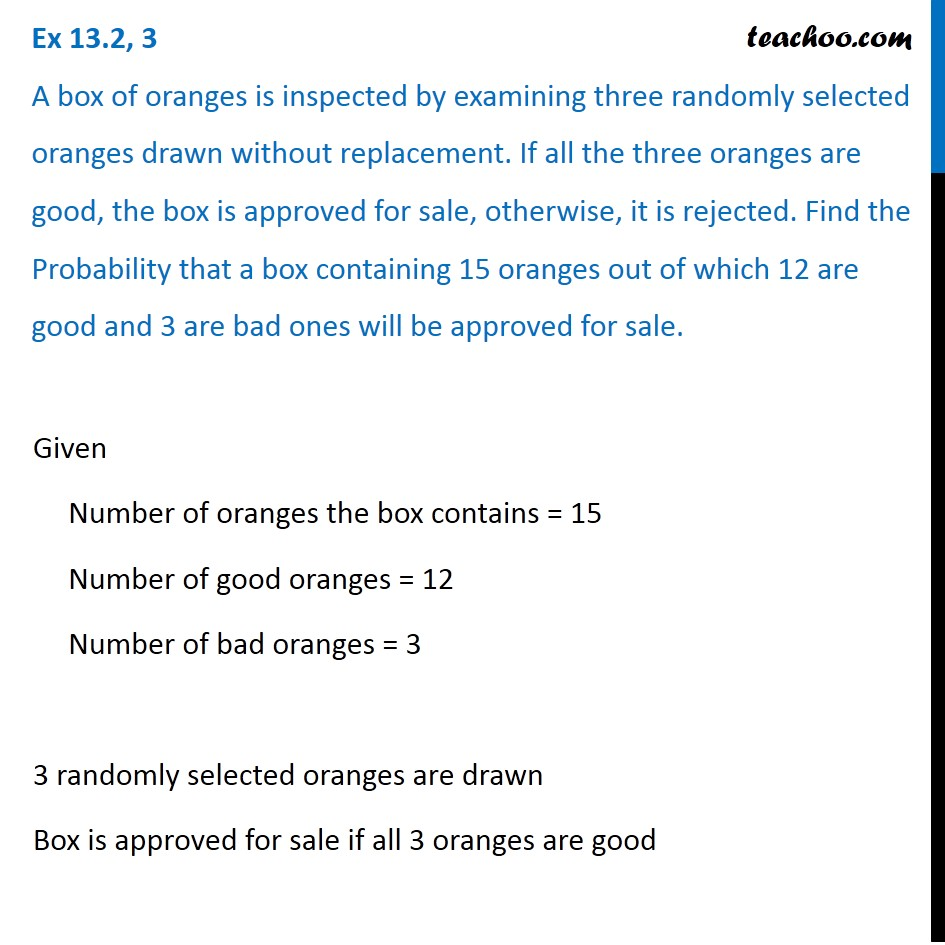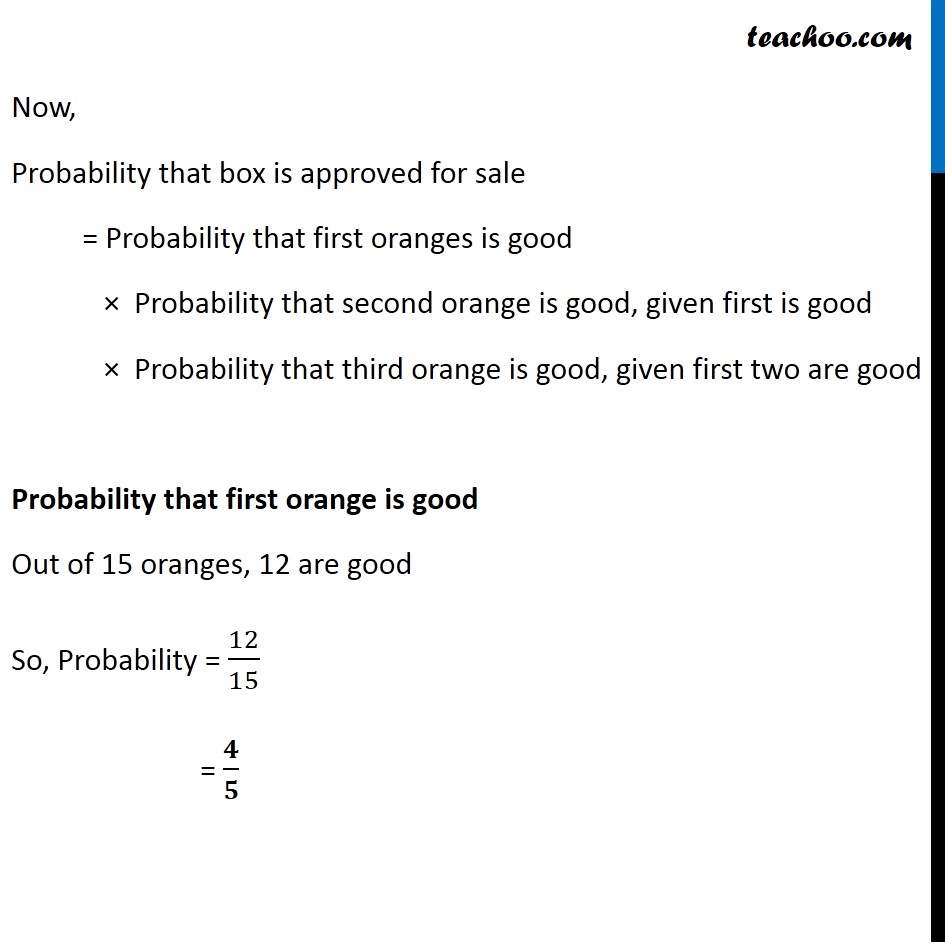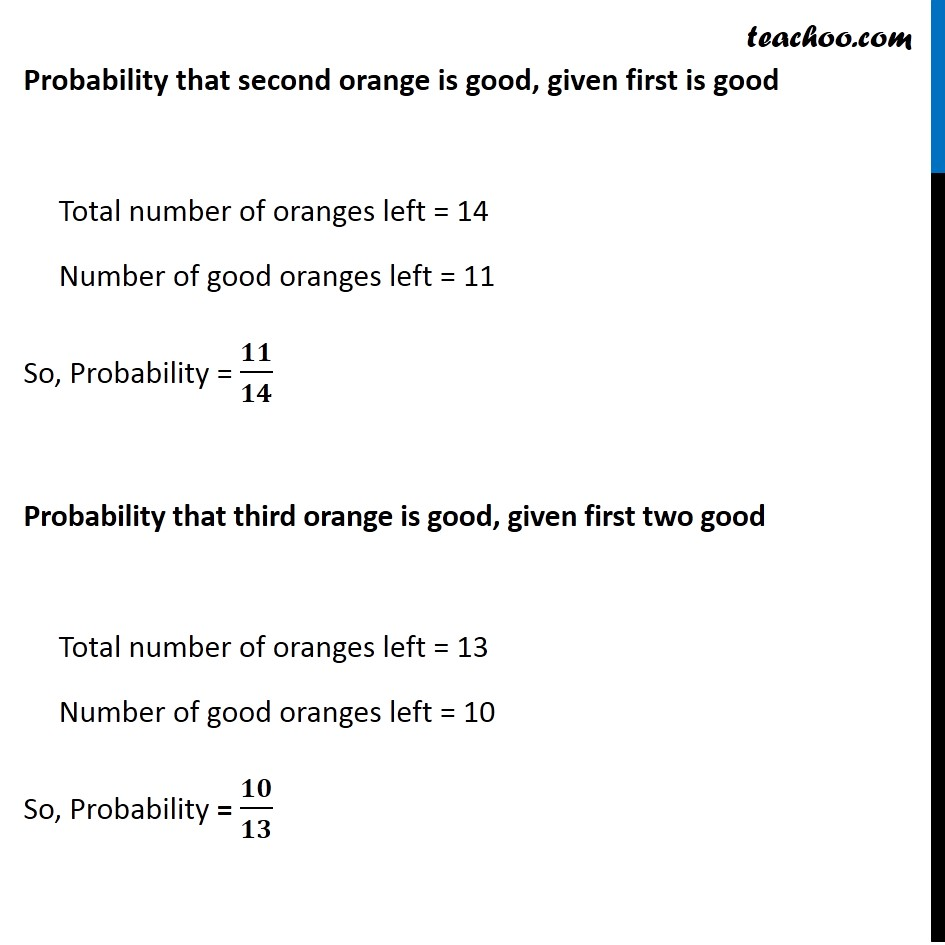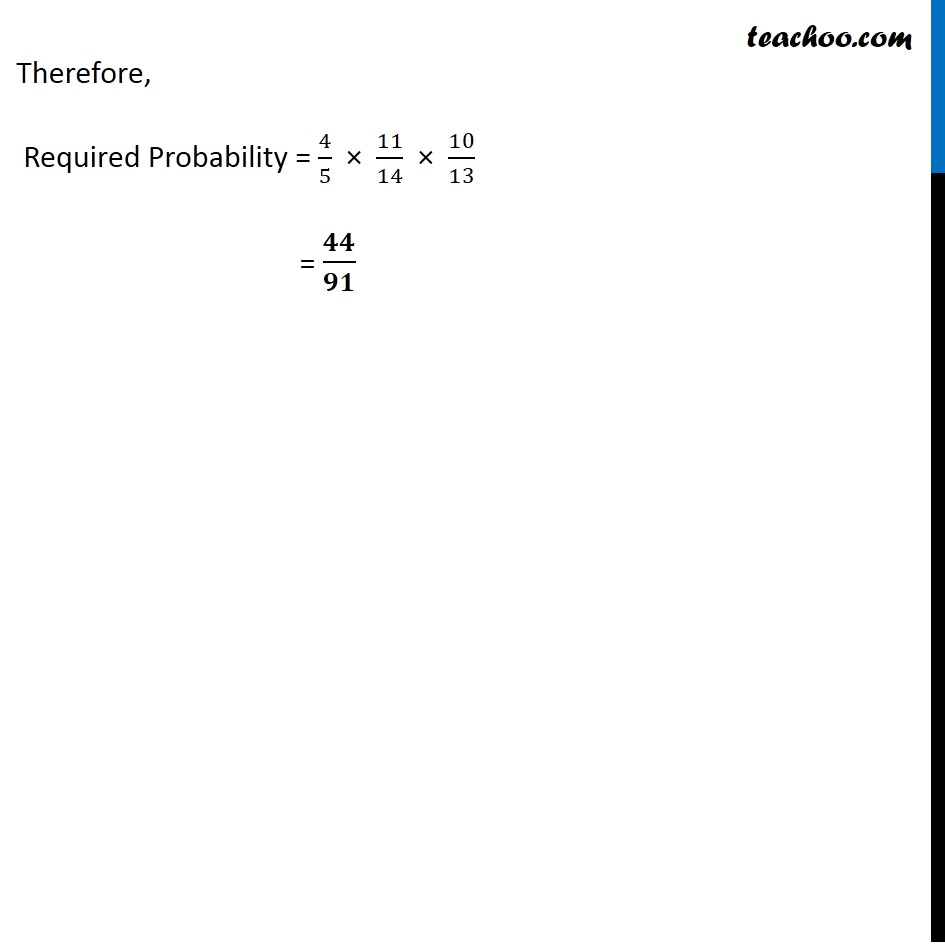Basic Probability

Chapter 13 Class 12 Probability
Concept wiseIntroducing your new favourite teacher - Teachoo Black, at only ₹83 per month

### Transcript

Ex 13.2, 3 A box of oranges is inspected by examining three randomly selected oranges drawn without replacement. If all the three oranges are good, the box is approved for sale, otherwise, it is rejected. Find the Probability that a box containing 15 oranges out of which 12 are good and 3 are bad ones will be approved for sale.Given Number of oranges the box contains = 15 Number of good oranges = 12 Number of bad oranges = 3 3 randomly selected oranges are drawn Box is approved for sale if all 3 oranges are good Now, Probability that box is approved for sale = Probability that first oranges is good × Probability that second orange is good, given first is good × Probability that third orange is good, given first two are good Probability that first orange is good Out of 15 oranges, 12 are good So, Probability = 12/15 = 𝟒/𝟓 Probability that second orange is good, given first is good Total number of oranges left = 14 Number of good oranges left = 11 So, Probability = 𝟏𝟏/𝟏𝟒 Probability that third orange is good, given first two good Total number of oranges left = 13 Number of good oranges left = 10 So, Probability = 𝟏𝟎/𝟏𝟑 Therefore, Required Probability = 4/5 × 11/14 × 10/13 = 𝟒𝟒/𝟗𝟏